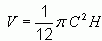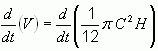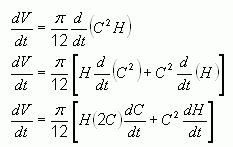SEARCH HOMEMath Central Quandaries & QueriesQuestion from Christy, a student: How do I go about answering this question, I know I have to find dv/dt, but I'm not sure how to start.  Thank you guys so much for helping me previously. The volume of a certain tree is given by V= 1/12pie C^2h where C is the circumference of the tree at the ground level and h is the height of the tree.  If C=5feet and growing at the rate of 0.2feet per yer, and if h=22feet and is growing at 4 feet per year, find the rate of growth of the volume, V.Hi Christy.

You are starting with the relationship:So the first step is to take the derivative with respect to time t on each side of the equation:Now do the derivation, first using the product rule:The last step is to substitute in the known values.

You are asked to find dV/dt when C is 5 ft, dC/dt is 0.2 ft/yr and dH/dt is 4 ft/yr.

So now you can just substitute these in to find your answer.

Cheers,
Stephen La Rocque and Harley Weston.Math Central is supported by the University of Regina and The Pacific Institute for the Mathematical Sciences.